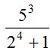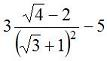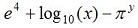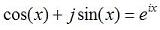# Examples: Basic Matlab Codes

 Below you can find examples on different types of arithmetic, exponential, trigonometry and complex number operations handled easily with MATLAB codes. To code this expression:, you can write the following instruction in the Matlab command window (or within an m-file):

>> 5^3/(2^4+1)

ans =

7.3529

To compute this formula:, you can always break down the commands and simplify the code (a final value can be achieved in several ways).

>>numerator = 3 * (sqrt(4) - 2)
numerator =

0

>>denominator = (sqrt(3) + 1)^2
denominator =

7.4641

>>total = numerator/denominator – 5

total =

-5

The following expression:, can be achieved as follows (assuming that x and y have values already):

>> exp(4) + log10(x) - pi^y

The basic MATLAB trigonometric functions are 'sin', 'cos', 'tan', 'cot', 'sec', and 'csc'. The inverses, are calculated with 'asin', 'atan', etc. The inverse function 'atan2' takes two arguments, y and x, and gives the four-quadrant inverse tangent. Angles are in radians, by default.

The following expression:, can be coded as follows (assuming that x has a value already):

>> (sin(pi/2))^2 + tan(3*pi*x).

MATLAB recognizes the letters i and j as the imaginary number. A complex number 4 + 5i may be input as 4+5i or 4+5*i in MATLAB.  The first case is always interpreted as a complex number, whereas the latter case is taken as complex only if i has not been assigned any local value.

Can you verify in MATLAB this equation (Euler's Formula)?
You can do it as an exercise!From 'Matlab Codes' to home

From 'Matlab Codes' to 'Matlab Examples'